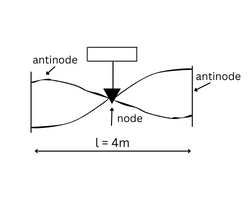Filter By

## All Questions

#### Consider  where [.] represents the greatest integer function. If f(x) is continuous at x=0, then  is equal toOption: 1 1Option: 2 2Option: 3 0Option: 4 -1

For x=0 and x=0+h ;[x+3]=3

For left hand limit to exist, we must have a+3=0

For continuity, we have

## Crack CUET with india's "Best Teachers"

• HD Video Lectures
• Unlimited Mock Tests
• Faculty Support#### If   stands for  the sum of given series where n is an even positive integer, is Option: 1 0Option: 2 Option: 3 Option: 4

We have

Therefore the value of given expression

## JEE Main high-scoring chapters and topics

Study 40% syllabus and score up to 100% marks in JEE

#### The set of points where the function    is differentiable isOption: 1 ROption: 2 Option: 3 Option: 4

Since |x-1| is not differentiable at x=1. So.    is not differentiable at x=1. Hence, the requried set is R-|1|.

#### A steel rod of length 400 cm is clamped at the middle. The frequency of the fundamental mode for the longitudinal vibrations of the rod is  2.5K Hz . Find the speed of sound in steel.Option: 1 20km/sOption: 2 25km/sOption: 3 15km/sOption: 4 26km/sdistance between two connected antinode

frequency of fundamental mode is

## NEET 2024 Most scoring concepts

Just Study 32% of the NEET syllabus and Score up to 100% marks

= curl =

\

## Crack CUET with india's "Best Teachers"

• HD Video Lectures
• Unlimited Mock Tests
• Faculty Support#### Locus of the midpoint of any focal chord of is  Option: 1  Option: 2  Option: 3  Option: 4

Let the midpoint be . Equation of this chord is . i.e., . It must pass through

. Thus required locus is

Hence option 2 is correct.

#### If at t=0, a travelling wave pulse on a string is described by the function  . What will be the wave function representing the pulse at time t, if the pulse is propagating along positive x-axis with speed 4m/s?Option: 1 Option: 2 Option: 3 Option: 4

If v is the speed of the wave, then